## Mathematics Paper 2 Questions and Answers - Momaliche Post Mock 2020 Exam

INSTRUCTIONS TO CANDIDATES

• This paper consists of two sections: Section I and Section II.
• Answer ALL questions from section I and ANY FIVE from section II
• All answers and workings must be written
• Non – Programmable silent electronic calculators and KNEC mathematical tables may be
used, except where stated otherwise.

SECTION I:
Answer all questions from this section

1. Use logarithm tables to evaluate (4 Marks)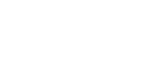2. Solve for x in the equation 2Sin2x − 1 = Cos2x + Sinx for 0°≤x≤360°      (3 Marks)
3.
1. Expand (1+3/x)5 upto the fifth term    (2 Marks)
2. Hence use your expansion to evaluate the value of (2.5)5 to 3 d.p. (2 Marks)
4. Make p the subject of the formula (3 Marks)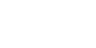5. The figure below shows a rectangular based right pyramid. Find the angle between the planes ABCD and ABV. (2marks)6. A object A of area 10cm2 is mapped onto its image B of area 60cm2 by a transformation whose matrix is given by P =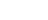. Find the possible values of x (3 Marks)
7. The position vectors of A and B are a = 4i + 4j – 6k and b = 10i + 4j + 12k. D is a point on AB such that AD:DB is 2:1. Find the co-ordinates of D (3 Marks)
8. A dealer has two types of grades of tea, A and B. Grade A costs Sh. 140 per kg. Grade B costs Sh. 160 per kg. If the dealer mixes A and B in the ratio 3:5 to make a brand of tea which he sells at Sh. 180 per kg, calculate the percentage profit that he makes (3 marks)
9. A variable Z varies directly as the square of X and inversely as the square root of Y. Find the percentage change in Z if X increased by 20% and Y decreased by 19% (3 Marks)
10. By rounding each number to the nearest tens, approximate the value of
2454 x 396
66
Hence calculate the percentage error arising from this approximation to 4 significant figures (3 Marks)
11. Find the centre and radius of the circle whose equation is 2x2 + 2y2 – 8x + 12y – 2 = 0
(3 Marks)
12. In the figure below AB = 8cm and O is the centre of the circle. Determine the area of the circle if angle OAB= 15° (3 Marks)13. Pipe A can fill a tank in 2 hours; pipes B and C can empty the tank in 5 hours and 6 hours respectively. How long would it take:
1. To fill the tank if A and B are left open and C closed (2 Marks)
2. To fill the tank with all the pipes open (2 Marks)
14.
1. Find the inverse of the matrix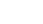(1 Mark)
2. Hence solve the simultaneous equation below using matrix method (3 Marks)
4x + 3y = 6
5y + 3x – 5 = 0
15. Evaluate by rationalizing the denominator and leaving your answer in surd form.(2 Marks)
√8
1+Cos 45°
16. Form the three inequalities that satisfy the given region R (3 Marks)SECTION II – 50 MARKS
Answer any FIVE questions from this section

1.
1. P, Q and R are three quantities such that P varies directly as the square of Q and inversely as the square root of R.
1. Given that P = 12 when Q = 24 and R = 36, find P when Q =27 and R = 121.
(3 Marks)
2. If Q increases by 10% and R decreases by 25%, find the percentage increase in P.     (4 marks)
2. If Q is inversely proportional to the square root of P and P = 4 when Q = 3. Calculate the value of P when Q = 8. (3 marks)
2.
1. complete the table for the curves y = 3sin (2x + 30°) and y = Cos2x ,use the range
0 ≤ x ≤ 1800
 x 0 15 30 45 60 75 90 105 120 135 150 165 180 y=3sin  (2x+30) 1.5 3 1.5 −1.5 −2.60 −1.00 1.5 y=Cos 2x 1 0 −0.866 −0.866 −0.5 0.866 1
2. Using the scale Horizontal axis 1cm represent 30°, vertical axis 1cm represent 1 unit, draw the graphs of y = 3 Sin (2x + 30) and y = Cos 2x (4 Marks)
3. Use your graph to solve the equation 3Sin (2x + 30) = Cos 2x (1 Mark)
4. Determine the following from your graph
1. Amplitude of y = 3Sin (2x + 30) (1 Mark)
2. Period of y = 3 Sin (2x + 30) (1 Mark)
3. Period of y = Cos 2x (1 Mark)
3. The 2nd and 5th terms of an arithmetic progression are 8 and 17 respectively. The 2nd, 10th and 42nd terms of the A.P. form the first three terms of a geometric progression. Find:
1. The 1st term and the common difference. (3 Marks)
2. The first three terms of the G.P and the 10th term of the G.P. (4 Marks)
3. The sum of the first 10 terms of the G.P. (3Marks)
4. The probability of passing KCSE depends on the performance in the KCPE. If the candidate passes the KCPE, the probability of passing KCSE is 4/5. If the candidate fails in the KCPE, the probability of passing KCSE is 3/5. If a candidate passes KCSE the probability that he/she will get employed is 5/8. If he/she fails KCSE the probability of getting employed is 1/3. The probability of passing KCPE is 2/3.
1. Draw a well labelled tree diagram to represent the above information. (2 Marks)
2. Using the tree diagram, find the probability that a candidate:-
1. Passes the KCSE (2 Marks)
2. Gets employed (2 Marks)
3. Passes KCSE and get employed (2 Marks)
4. Passes KCPE and does not get employed (2 Marks)
5. The heights of 100 maize plants were measured to the nearest centimeter and the results recorded in the table shown below.
 Height x (cm) Frequency d d2 fd fd2 cf 25-29 5 −15 5 30-34 12 −24 17 35-39 18 −1 1 −18 35 40-44 30 0 0 0 65 45-49 17 1 1 50-54 11 2 55-59 7 3

1. Complete the table (2 Marks)
2. Calculate to 2 d.p.
1. The mean (2 Marks)
2. The standard deviation (2 Marks)
3. Using the data above plot an ogive and use it to find the quartile deviation (4 Marks)
6. The figure below shows rectangular plot ABCD with AB =60m and BC=45m. PN is a vertical pole of length 30m to which four taut wire PB1, PC1,PD and PA are attachedCalculate
1. length of the projection of PCon the plane ABCD (2mrks)
2. the angle PC made with the base ABCD (3mks)
3. The angle between the planes PBC and ABCD (3Mrks)
4. If point A is to be the North of point C. calculate the bearing of B from A (2mks)
7.
1. Construct a parallelogram ABCD in which AB = 9cm, AD = 5cm and angle BAD = 60°. Measure the length AC (3 Marks)
2. Show the locus of point P which moves so that it is equidistant from A and C.(1 Mark)
3. Show the locus of point Q which moves such that angle BQD = 90°.(2 Marks)
4. The position of point X such that AX≥XC and angle BXD = 90°(2 Marks)
5. Shade the region inside the parallelogram such that AX≥XC and angle BXD≥90°
(2 Marks)
8.
1. Draw ∆PQR whose vertices are P(1,1), Q(-3,2) and R(0,3) on the grid provided
(1 Mark)
2. Find and draw the image P’Q’R’, image of ∆PQR under the transformation whose matrix is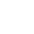(3 Marks)
3. P’Q’R’ is then transformed into P’’Q’’ R’’ by the transformation of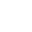matrix. Find the co-ordinates of P’’Q’’ R’’ and draw the image (3 Marks)
4. Describe fully the single transformation which maps PQR onto P’’Q’’ R’’. Find the matrix of this transformation     (3 Marks)## MARKING SCHEME

1.  No. Log 45.30.006978.450×10−1 0.8450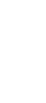−1−2 + 2.7808     3          3←T.9296
2. 2Sin2 x – 1 = 1 – Sin2x + Sinx
3Sin2x – Sinx – 2 = 0
(3Sinx + 2) (Sin x – 1) = 0
Either
Sinx = 0.6667 or Sin x = 1
x = 90°, 221.81, 318.19
3.
1. 1 + 5 x 3/x + 10 x (3/x)2+10 x (3/x)3+ 5 x (3/x)4….
1 + 15/x + 90/x2 + 270/x+ 405/x+ ….
2. (2.5)2 = (1+ 3/x)5
1.5 = 3/x
x = 2
1 + 15/2 + 90/4 + 270/8 + 405/16
1 + 7.5 + 22.5 + 33.75 + 25.3125 + ….
=90.063
4.E2Y – 3E−2XP = P – 3u
P + 3E2XP = E2Y+3u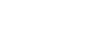5.Cos θ = 1.5
√60
= 0.1936
θ = Cos  0.1936
θ = 78.84°
6. A.S.F = Determinant of matrix
60/10 = x (x + 3) – 12
x2 + 3x – 12 = 6
x2 + 3x – 18 = 0
x2 – 3x + 6x – 18 = 0
x(x – 3x) + 6(x – 3) = 0
(x + 6) (x – 3) = 0
x = –6 or x = 3
7.8i + 4j + 6k
Co-ordinates of D are(8, 4, 6)
8. Cost price = 3 x 140+5 x 160
8
= 152.50
Profit = 180 – 152.50
= 27.50
% Profit = 27.50  x 100
152.50
= 18.03%

9.1.6z
% Change = 1.6Z-Z x 100%
Z
z (1.6-1) x 100
z
= 60%
10. 2450 x 400
70
= 14000
2454 x 396 = 14724
66
% error 14724-14000 x 100
14724
= 4.917
11. x2 – 4x + y2 + 6y – 1 = 0
x2 – 4x + 4 + y2 + 6y + 9 = 14
(x – 2)2 + (y + 3)2 = 14
Centre (2, -3)
12. ∠OBA = 15°
∠AOB = 180 – (15 + 15) = 150°
∠ACB = 75°
2R =     8
Sin 75
R =       8
2 X 09695
r = 4.146
Area of circle
3.142 x 4.1462
=54.01
13.
1. ½ −1/5 = 3/10
Required time 10/3
3 1/3 or 3 hrs 20 min
2. ½ − 1/51/6 = 4/30
Required time 30/4
=7½ hrs
1. Det. 20 – 9 = 11
Inverse = 1/11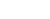2.x = 15/11, y = 2/11
14.      √8
1+Cos 45°
2√2
1+ 1/√22√2−2
½
2(√2−1)
= 4(√2 −1)
½
15. L1 y = x
y > x

L2 x/4 + y/4 = 1
x + y = 4
x + y ≤ 4

L3 x/-1 + y/3 = 1
−3x + y = 3
y – 3x = 3
y – 3x ≤ 3

1.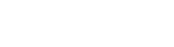1. K = P√R = 12 ×√361/8
Q2         242
Hence P = 1   Q2
8  √R
When Q = 27, R = 121
P =1/8 ×   272 = 729
√121    88
2. Q1 = 1.21Q2
R1 = 0.866025403√R
P1 = K ×  1.21Q² = 1.397187651 K  Q2
√0.75R                            √R
New value of New change =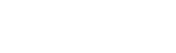=39.7187651%
Hence increase of 39.72%
2. Q = K 1/√P
K = Q√P
=3√4
=6 Eqn = Q = 6 1/√P
P = (K1/Q)2
=(6 × 1/8)2 = (6/8)2
= 9/16

16.17.
1. a + d = 8
a + 4d= 17
3d=9
d = 3
∴ a = 5
2. 2nd = 8
10th = 5 + 9 × 3 = 32
42nd = 5 + 41 × 3 = 128
∴ GP is 8, 32, 128, - - - -
a = 8
r = 4
nth term of G.P = arn–1
∴ 10th term = 8(4)9
= 2097152
3.8/3 × 1048575
= 2796200
1.2.
1. (2/3 × 4/5) + (1/3 × 3/5)
8/15+3/15
11/15
2. (2/3 × 4/5 × 5/8) + (2/3 × 1/5 × 1/3) + (1/3 × 3/5 × 5/8) + (1/3 × 3/5 × 1/3) = 197/360
3. (2/3 × 4/5 × 5/8) + (1/3 × 3/5 × 5/8) = 11/24
4. (2/3 × 4/5 × 3/8) + (2/3 × 1/5 × 2/3) = 13/45
18.
1.
 Height x (cm) Frequency d d2 fd fd2 cf 25-29 5 −3 9 −15 45 5 30-34 12 −2 4 −24 48 17 35-39 18 −1 −1 −18 18 35 40-44 30 0 0 0 0 65 45-49 17 1 1 17 17 82 50-54 11 2 4 22 44 93 55-59 7 3 9 21 63 100 ∑f =100 ∑fd =3 ∑fd2 =235
2.19.
1. AC = √602 + 452
=75
NC = ½AC
= 37.5m
2.tan r = 30
37.5
r = tan−130/37.5)
= 38.66°
3.tan r = 30/30
=45°
4.n2 = a2 + b2 − 2ab cos n
ba2 = 37.52 cos N
cos−1 = −0.28
∠N = 106.3°
therefore the bearing of B from A = 360 − 106.3
= 253.7°
20.21.
1.2.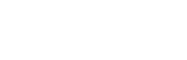3.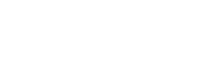Coordinates PII(2,2), QII(−6,4), RII(0,6)
4. It is an enlargement centre origin (0,0) scale factor 2Note correct the 2nd matrix (b) from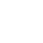to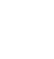• ✔ To read offline at any time.
• ✔ To Print at your convenience
• ✔ Share Easily with Friends / Students